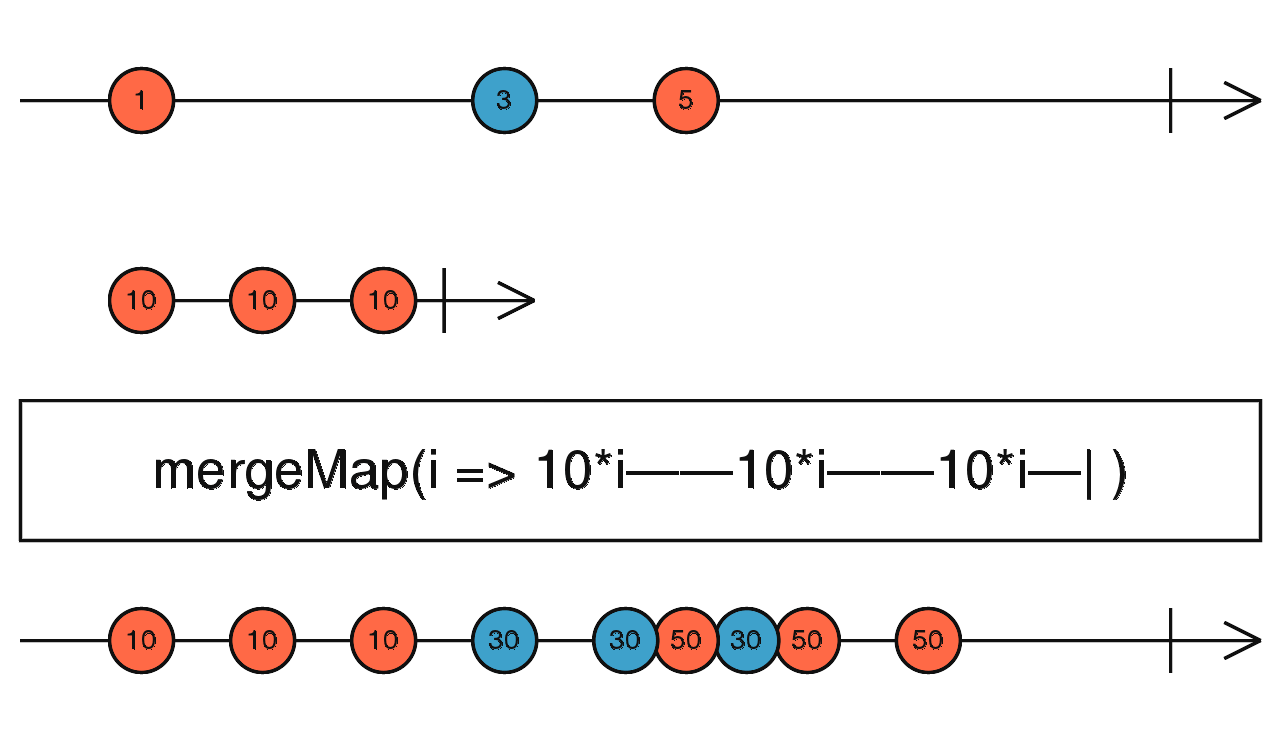/RxJS

# flatMap

`function` `stable`

Projects each source value to an Observable which is merged in the output Observable.

### `flatMap<T, R, O extends ObservableInput<any>>(project: (value: T, index: number) => O, resultSelector?: ((outerValue: T, innerValue: ObservedValueOf<O>, outerIndex: number, innerIndex: number) => R) | number, concurrent: number = Number.POSITIVE_INFINITY): OperatorFunction<T, ObservedValueOf<O> | R>`

#### Parameters

 project A function that, when applied to an item emitted by the source Observable, returns an Observable. resultSelector Optional. Default is `undefined`. Type: `((outerValue: T, innerValue: ObservedValueOf, outerIndex: number, innerIndex: number) => R) | number`. concurrent Optional. Default is `Number.POSITIVE_INFINITY`. Maximum number of input Observables being subscribed to concurrently.

#### Returns

`OperatorFunction<T, ObservedValueOf<O> | R>`: An Observable that emits the result of applying the projection function (and the optional deprecated `resultSelector`) to each item emitted by the source Observable and merging the results of the Observables obtained from this transformation.

## Description

Maps each value to an Observable, then flattens all of these inner Observables using `mergeAll`.Returns an Observable that emits items based on applying a function that you supply to each item emitted by the source Observable, where that function returns an Observable, and then merging those resulting Observables and emitting the results of this merger.

## Example

Map and flatten each letter to an Observable ticking every 1 second

```import { of, interval } from 'rxjs';
import { mergeMap, map } from 'rxjs/operators';

const letters = of('a', 'b', 'c');
const result = letters.pipe(
mergeMap(x => interval(1000).pipe(map(i => x+i))),
);
result.subscribe(x => console.log(x));

// Results in the following:
// a0
// b0
// c0
// a1
// b1
// c1
// continues to list a,b,c with respective ascending integers```

### `mergeMap(project: (value: T, index: number) => O, concurrent?: number): OperatorFunction<T, ObservedValueOf<O>>`

#### Parameters

 project Type: `(value: T, index: number) => O`. concurrent Optional. Default is `undefined`. Type: `number`.

#### Returns

`OperatorFunction<T, ObservedValueOf<O>>`

### `mergeMap(project: (value: T, index: number) => O, resultSelector: undefined, concurrent?: number): OperatorFunction<T, ObservedValueOf<O>>`

#### Parameters

 project Type: `(value: T, index: number) => O`. resultSelector Type: `undefined`. concurrent Optional. Default is `undefined`. Type: `number`.

#### Returns

`OperatorFunction<T, ObservedValueOf<O>>`

### `mergeMap(project: (value: T, index: number) => O, resultSelector: (outerValue: T, innerValue: ObservedValueOf<O>, outerIndex: number, innerIndex: number) => R, concurrent?: number): OperatorFunction<T, R>`

#### Parameters

 project Type: `(value: T, index: number) => O`. resultSelector Type: `(outerValue: T, innerValue: ObservedValueOf, outerIndex: number, innerIndex: number) => R`. concurrent Optional. Default is `undefined`. Type: `number`.

#### Returns

`OperatorFunction<T, R>`